## ML Aggarwal Class 8 Solutions for ICSE Maths Chapter 18 Mensuration Ex 18.3

Question 1.
The volume of a cube is 343 cm3, find the length of an edge of cube.
Solution:
Volume of a cube = 343 cm3
Let a be the edge of cube, then
V = a3 = 343 = (7)3 .
∴ a = 7 cm

Question 2.
Fill in the following blanks: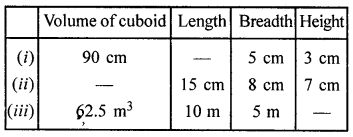Solution: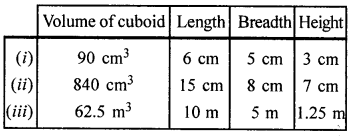Question 3.
Find the height of a cuboid whose volume is 312 cm3 and base area is 26 cm2.
Solution:
Volume of a cuboid = 312 cm3
Base area = l × b = 26 cm2
∴ Height= $$\frac{\text { Volume }}{\text { Base area }}=\frac{312}{26}$$ = 12cm

Question 4.
A godown is in the form of a cuboid of measures 55 m × 45 m × 30 m. How many cuboidal boxes can be stored in it if the volume of one box is 1.25 m3?
Solution:
Length of a godown (l) = 55 m
Height (h) = 30 m
Volume = lbh = 55 × 45 × 30 m3
Volume of one box = 1.25 m3
Number of box = $$\frac{74250}{1.25}$$ = 59400 boxes

Question 5.
A rectangular pit 1.4 m long, 90 cm broad and 70 cm deep was dug and 1000 bricks of base 21 cm by 10.5 cm were made from the earth dug out. Find the height of each brick.
Solution:
Here l = 1·4 m = 140 cm, b = 90 cm, h = 70 cm
Volume of rectangular pit = l × b × h
= (140 × 90 × 70) cm3 = 882000 cm3
Volume of brick = 21 × 10.5 × hQuestion 6.
If each edge of a cube is tripled, then find how many times will its volume become?
Solution:
Let edge of a cube = x
Then volume = x3
If the edge is trippled, then
Edge = 3x
Now, volume = (3x)3 = 27x3
∴ Its volume is 27 times the volume of the given cube.

Question 7.
A milk tank is in the form of cylinder whose radius is 1.4 m and height is 8 m. Find the quantity of milk in litres that can be stored in the tank.
Solution:
Radius of the milk cylindrical tank = 1.4 m and height (h) = 8 m
∴ Volume of milk in the tank = πr2h
= $$\frac{22}{7}$$ × 1.4 × 1.4 × 8 m3
= 49.28 m3
= 49.28 × 1000 litres
= 49280 litres

Question 8.
A closed box is made of 2 cm thick wood with external dimension 84 cm × 75 cm × 64 cm. Find the volume of the wood required to make the box.
Solution:
Thickness of the wood used in a closed box = 2 cm
External length of box (L) = 84 cm
and height (h) = 64 cm
∴ Internal length (l) = 84 – (2 × 2) = 84 – 4 = 80 cm
Breadth (b) = 75 – (2 × 2) = 75 – 4 = 71 cm
and height (h) = 64 – (2 × 2) = 64 – 4 = 60 cm
∴ Volume of wood used
= 84 × 75 × 64 – 80 × 71 × 60 cm3
= 403200 – 340800 cm3
= 62400 cm3

Question 9.
Two cylindrical jars contain the same amount of milk. If their diameters are in the ratio 3 : 4, find the ratio of their heights.
Solution:
Ratio in diameters of two cylindrical jars = 3 : 4
But their volume is same.
Let h1 and h2 be the heights of the two jars respectively.
Let radius of the first jar (r1) = $$\frac{3 x}{2}$$
and radius of the second jar (r2) = $$\frac{4 x}{2}$$
According to the condition,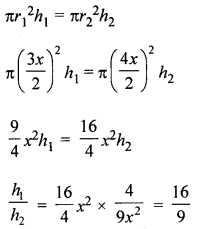∴ Ratio in their heights =16 : 9

Question 10.
The radius of the base of a right circular cylinder is halved and the height is doubled. What is the ratio of the volume of the new cylinder to that of the original cylinder?
Solution:
Let radius of a cylinder = r
and height = h
Volume = πr2h
Its radius is halved and height is doubled, then∴ Ratio in the volumes of the new cylinder to old one
= $$\frac{\pi r^{2} h}{2} : \pi r^{2} h$$ = 1 : 2

Question 11.
A rectangular piece of tin of size 30 cm × 18 cm is rolled in two ways, once along its length (30 cm) and once along its breadth. Find the ratio of volumes of two cylinders so formed.
Solution:
Size of rectangular tin plate = 30 cm × 18 cm
(i) When rolled along its length (30 cm),
then Circumference of the circle so formed = 30 cm
Circumference = 2πr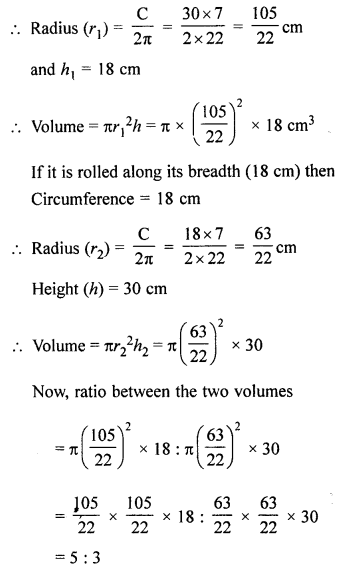Question 12.
Water flows through a cylindrical pipe of internal diameter 7 cm at 5 m per sec. Calculate
(i) the volume in litres of water discharged by the pipe in one minute.
(ii) the time in minutes, the pipe would take to fill an empty rectangular tank of size 4 m × 3 m × 2.31 m.
Solution:
Speed of water flow through cylindrical pipe = 5 m/sec.
Internal diameter of the pipe = 7 cm
∴ Radius (r) = $$\frac{7}{2}$$cm
∴ Length of water flow in 1 minutes (h)
= 5 × 60 = 300 m
∴ Volume of water = πr2h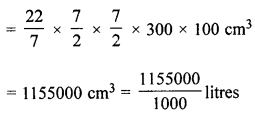= 1155 litres
Now volume of water = 1155000 cm3
Volume of rectangular tank of size
= 4m × 3m × 2.31m
= 27.72 m3
Speed of water 4 m/sec.
Radius of pipe = $$\frac{7}{2}$$ cm
Volume of water in 1 sec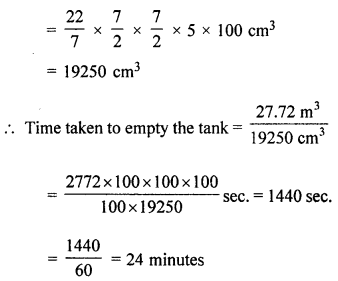Question 13.
Two cylindrical vessels are filled with milk. The radius of one vessel is 15 cm and height is 40 cm, and the radius of other vessel is 20 cm and height is 45 cm. Find the radius of another cylindrical vessel of height 30 cm which may just contain the milk which is in the two given vessels.
Solution:
Radius of one cylinder (r1) = 15 cm
and height (h1) = 40 cm
and radius of second cylinder (r2) = 20 cm
and height (h2) = 45 cm∴ Radius of the third cylinder = 30 cm

Question 14.
A wooden pole is 7 m high and 20 cm in diameter. Find its weight if the wood weighs 225 kg per m3 .
Solution:
Height of pole (h) = 7 m
Diameter = 20 cm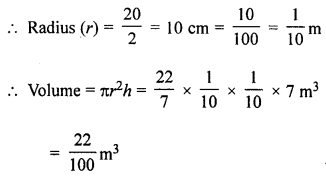Weight of wood = 225 kg per m3
Total weight = 225 × $$\frac{22}{100}=\frac{99}{2}$$ = 49.5 kg

Question 15.
A cylinder of maximum volume is cut from a wooden cuboid of length 30 cm and cross-section a square of side 14 cm. Find the volume of the cylinder and the volume of the wood wasted.
Solution:
A cylinder of the maximum volume is cut from a wooden cuboid
of length 30 cm and cross-section a square side 14 cm.∴ Diameter of the cylinder = 14 cm
∴ Radius (r) = $$\frac{14}{2}$$ = 7 cm
and height (h) = 30 cm
Volume of cuboid = 30 × 14 × 14 = 5880 cm3
Volume of cylinder = πr2h
= $$\frac{22}{7}$$ × 7 × 7 × 30 = 4620 cm3
and wastage of wood = 5880 – 4620 = 1260 cm3